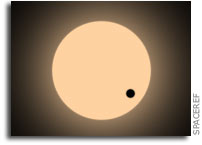# The Mass-Radius Relation Between 60 Exoplanets Smaller than 4 Earth RadiiWe study the masses and radii of 60 exoplanets smaller than 4 Earth radii with orbital periods shorter than 100 days.

We find a nearly linear mass-radius relation: M/M_E= 3.17 (R/R_E)^0.87, which is a shallower power-law index than in many previous mass-radius relations. The RMS of planet masses to this fit is 3.9 Earth masses, and our best fit has reduced chi squared = 3.1, indicating a diversity in planet compositions below 4 Earth radii. Fitting density vs. radius with a polynomial, we find planet density = 11.50 - 5.97 (R/R_E) + 0.84 (R/R_E)^2. The mass-radius and mass-density relations reflect that planet density decreases as radius increases, indicating that larger exoplanets have a significant fraction of volatiles by volume (such as H/He envelopes).

Exoplanets have densities comparable to that of Earth at R = 1.5 R_E, indicating likely rocky compositions among planets smaller than 1.5 Earth radii. The scaling of the mass-radius relationship for exoplanets smaller than 1.5 Earth radii is not well-constrained but if we include the solar system terrestrial planets, we find that a relationship of M/M_E = 1.08 (R/R_E)^3.45 is a significant improvement over the nearly linear relationship.

Lauren M. Weiss, Geoffrey W. Marcy (Submitted on 3 Dec 2013)